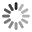The best jokes and joke writers!## Log Negative Zero

Theorem: log(-1) = 0 Proof: a. log[(-1)^2] = 2 * log(-1) On the other hand: b. log[(-1)^2] = log(1) = 0 Combining a) and b) gives: 2* log(-1) = 0 Divide both sides by 2: log(-1) = 0

## Bermuda Triangle

Q: Why do so many people die from heat exhaustion when traveling through the Bermuda Triangle?

A: Because it's 180 degrees inside a triangle.

## All Numbers Equal

Theorem: All numbers are equal.

Proof: Choose arbitrary a and b, and let t = a + b. Then a + b = t(a + b)(a - b) = t(a - b)a^2 - b^2 = ta - tba^2 - ta = b^2 - tba^2 - ta + (t^2)/4 = b^2 - tb + (t^2)/4(a - t/2)^2 = (b - t/2)^2a - t/2 = b - t/2a = b

So all numbers are the same, and math is pointless.

## Highest Number

One day Jimmy went up to his father and asked, "Daddy, what's the highest number?" His daddy said, "Well, I'm not exactly sure, but I think it's in the stimulus package."

## Movie Ratings

Just saw a pirated movie

On a scale of 1-10, I'd give a 3.14